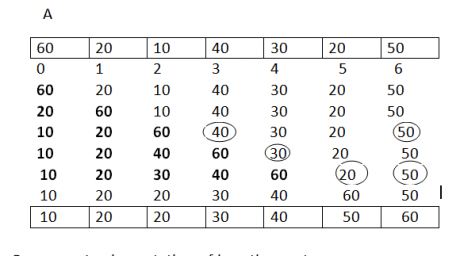# Insertion sort in Data Structures

21 September, 2018

Ratings

## Insertion sort

Suppose an array ‘A’ with ‘n’ elements A. A910,A,---------A[N-1] is in memory. The insertion sort algorithm in data structures scans ‘A; from A to A[N-1] insert each element A[k] into its proper position in the previously sorted sub array A,A,A---- A[K]                      That is pass1                   A by itself ip trivially sorted. pass2                   A is inserted either before or after aA So that : A,A is sorted pass3: A is inserted into its proper place in A A, that is before A between A A or after A, so that : A,A,A is sorted pass4:  is inserted into its proper place in A,A so that : A,A,A,A is sorted. PassN : A[N] is inserted into its proper place in A,A,-------A[N-1] so that : A,A--- is sorted.   Example :### Insertion Sort Program

Implementation of insertion sort in data structures # include <stdio.h> # include <conio.h> void main() { int n,i,j,a,temp; void insertion sort(int  a,int n); clrscr(); printf(“Enter n value: ”); scanf(“%d”,&n); printf(“Enter the elements:\n”); for(i=0; i<n;i++) { scanf(“%d”,&a[i]); } Insertion sort(a,n); } insertion sort(a,n); printf(“The sorted elements are:\n”); for(i=0;i<n;i++) { printf(“%5d”,a[i]); } } void insertion sort (int a, int n) { int i,j,temp; for(i=1;i<n;i++) { if(a[j]<a[j-1]) { temp=a[j]; a[j]=a[j-1]; a[j-1]=temp; } } } }

### output :

Enter ‘n’ value : 5 Enter the elements:     2       4       3       1       5 The elements are :  2   3       4       5     Time complexity :

 Algorithm Worst case Average case Insertion sort n(n+1)/2=o(n2) n(n-1)/4=o(n2)